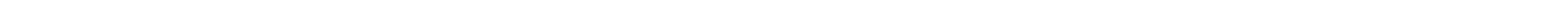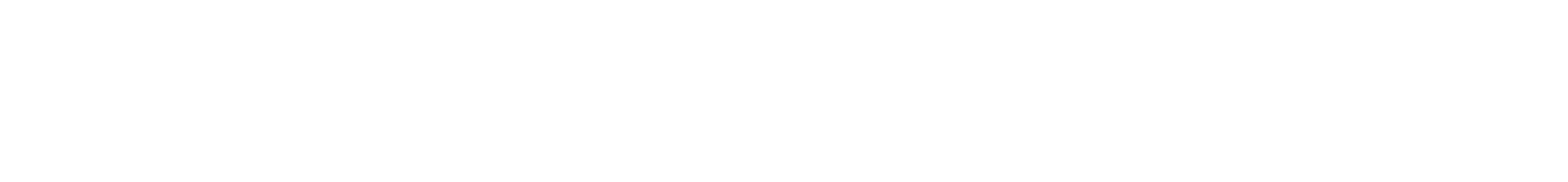```===================Example 1 ===================//  reciprocal.c//  This example shows how to //    (1)  Ask the user for a "floating point" number //    (2)  get the number//    (3)  Create your own function//    (4)  Print a floating point number//  When you try this program, test it out with various numbers.  At least try//  0.25    -- super simple//  3    -- watch what happens with a repeating decimal//  2e25 -- a huge number.  2x10^25//  2e-25  -- a tiny number//  Also try typing in nonsense, such as "hello"//  Compile command for this code c version://  gcc -Wall -W -Werror reciprocal.c -o reciprocal//  Compile command for this code c++ version://  g++ -Wall -W -Werror reciprocal.c -o reciprocal// To run the program type:// ./reciprocal//  More info:  "double" tells the compiler that we want decimal number.  Not integers.#include <stdio.h>//  Create a new function which will compute the reciprocal of a number.double reciprocal(double x)   // x is the input{  double a;                   // a is the output  a = 1/x;  return(a);}int main(){  double your_number;  double the_reciprocal;  printf("Give me a number: ");  scanf("%lf",&your_number);     // "&" is used because its the memory address of "your_number" that matters  // "%lf" means that the user will type in a "floating" style number (a decimal)  // See http://www.cs.utah.edu/~zachary/isp/tutorials/io/io.html  printf("OK, you gave me %g\n",your_number);  // Always use %g for displaying "double" numbers  the_reciprocal = reciprocal(your_number);  printf("The reciprocal is %g\n",the_reciprocal);  printf("The reciprocal, with more precision, is %2.18g\n",the_reciprocal);  return(0);}===================Example 2 ===================//  reciprocal_for_dummy.c//  This example shows how to //    (1)  Ask the user for a "floating point" number //    (2)  get the number//    (3)  Create your own function//    (4)  Print a floating point number//  When you try this program, test it out with various numbers.  At least try//  0.25    -- super simple//  3    -- watch what happens with a repeating decimal//  2e25 -- a huge number.  2x10^25//  2e-25  -- a tiny number//  Also try typing in nonsense, such as "hello"//  Compile command for this code c version://  gcc -Wall -W -Werror reciprocal_for_dummy.c -o reciprocal_for_dummy//  Compile command for this code c++ version://  g++ -Wall -W -Werror reciprocal_for_dummy.c -o reciprocal_for_dummy// To run the program type:// ./reciprocal_for_dummy//  More info:  "double" tells the compiler that we want decimal number.  Not integers.#include <stdio.h>//  Create a new function which will compute the reciprocal of a number.double reciprocal_for_dummy(double x)   // x is the input{  double a;                   // a is the output  a = 1/x;  return(a);}int main(){  double your_number;  double new_number;  int OK_number;  do {    printf("Give me a number: ");    OK_number = scanf("%lf",&your_number); // If the user typed in an OK number, then OK_nummber will be 1    // "&" is used because its the memory address of "your_number" that matters    // "%lf" means that the user will type in a "floating" style number (a decimal)    // See http://www.cs.utah.edu/~zachary/isp/tutorials/io/io.html    // printf("OK_number=%d\n",OK_number);    if (OK_number != 1) {      printf("You dummy.  Give me a valid number!!!\n");       scanf ("%*[^\n]");  // Flush out all the junk that the dummy typed in.        //  See http://home.datacomm.ch/t_wolf/tw/c/getting_input.html    }  } while (OK_number != 1);  printf("OK, you gave me %g\n",your_number);  // Always use %g for displaying "double" numbers  new_number = reciprocal_for_dummy(your_number);  printf("The reciprocal is %g\n",new_number);  printf("The reciprocal, with more precision, is %2.18g\n",new_number);  return(0);}===================Example 3 ===================//  factorial_for_dummy.c//  This example shows how to //    (1)  Ask the user for an integer number //    (2)  get the number//    (3)  Create your own function - in this example the Factorial function. Factorial x is written x!//    Factorial is like this:  3!=2x3=6, 4!=2x3x4=24, 5!=2x3x4x5=120, etc.//    (4)  Print it out.//  When you try this program, test it out with various numbers.  At least try//  5//  16//  17   (You will notice that the result is wrong for 17.  Why?)//  Also try typing in nonsense, such as "hello"//  Try 0 and negative numbers.  Does it work?//  Compile command for this code c version://  gcc -Wall -W -Werror factorial_for_dummy.c -o factorial_for_dummy//  Compile command for this code c++ version://  g++ -Wall -W -Werror factorial_for_dummy.c -o factorial_for_dummy//  To run the program type://  ./factorial_for_dummy//#include <stdio.h>   // Include the standard input-output library.  Needed for keyboard.//  Create a new function which will compute the reciprocal of a number.int factorial(int x)   // x is the input{  int loop_count;  int a;                   // a is the output  a=1;  for (loop_count=2; loop_count < x+1;loop_count++) a=a*loop_count;  return(a);}int main(){  int your_number;  int new_number;  int OK_number;  do {    printf("Give me a number: ");    OK_number = scanf("%d",&your_number);   // Get the number from the keyboard    if (OK_number != 1) {      printf("You dummy.  Give me a valid number!!!\n");       scanf ("%*[^\n]");  // Flush out all the junk that the dummy typed in.        //  See http://home.datacomm.ch/t_wolf/tw/c/getting_input.html    }  } while (OK_number != 1);  printf("OK, you gave me %d\n",your_number);  // Use %d for displaying integer numbers  new_number = factorial(your_number);  printf("%d!=%d\n",your_number,new_number);  return(0);}===================Example 4 ===================// bits_in_a_number.c//  This program asks the user to type in a number, and then it prints it out in binary//  Compile command for this code c version://  gcc -Wall -W -Werror bits_in_a_number.c -o bits_in_a_number//  Compile command for this code c++ version://  g++ -Wall -W -Werror bits_in_a_number.c -o bits_in_a_number//  To run the program type://  ./bits_in_a_number#include <stdio.h>   // Include the standard input-output library.  Needed for keyboard and printing.int main(){  int your_number;  int bit_mask;   //Used to select individual bits.  It will be all zeroes, except for desired bit.  int bit_num;  int OK_number;  do {    printf("Give me a number: ");    OK_number = scanf("%d",&your_number);   // Get the number from the keyboard    if (OK_number != 1) {      printf("You dummy.  Give me a valid number!!!\n");       scanf ("%*[^\n]");  // Flush out all the junk that the dummy typed in.        //  See http://home.datacomm.ch/t_wolf/tw/c/getting_input.html    }  } while (OK_number != 1);  printf("OK, you gave me %d\n",your_number);  // Use %d for displaying integer numbers  for (bit_num=16; bit_num >= 0; bit_num--) {    bit_mask = 1 << bit_num;    printf("%d  ",bit_mask);    if ((bit_mask & your_number) == 0) printf("0"); else printf("1"); //bitwise "and".  Only the selected bit    //    comes through.      printf("\n");  }  printf("\n");  return(0);}
```